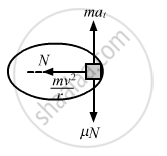Share

# A Block of Mass M Moves on a Horizontal Circle Against the Wall of a Cylindrical Room of Radius R. the Floor of the Room on Which the Block Moves is Smooth - Physics

ConceptCircular Motion

#### Question

A block of mass m moves on a horizontal circle against the wall of a cylindrical room of radius R. The floor of the room on which the block moves is smooth but the friction coefficient between the  wall and the block is μ. The block is given an initial speed v0. As a function of the speed v write (a) the normal force by the wall on the block, (b) the frictional force by wall and (c) the tangential acceleration of the block. (d) Integrate the tangential acceleration $\left( \frac{dv}{dt} = v\frac{dv}{ds} \right)$ to obtain the speed of the block after one revolution.

#### SolutionGiven:
Radius of the room = R
Mass of the block = m
(a) Normal reaction by the wall on the block = N = $\frac{m v^2}{R}$

(b) Force of frictional by the wall = $\mu N = \frac{\mu m v^2}{R}$

(c) Let at be the tangential acceleration of the block.
From figure, we get :

$- \frac{\mu m v^2}{R} = m a_t$

$\Rightarrow a_t = - \frac{\mu v^2}{R}$

(d) $\text { On using a }= \frac{dv}{dt} = v\frac{dv}{ds}, \text { we get : }$

$v\frac{dv}{ds} = \frac{\mu v^2}{R}$

$\Rightarrow ds = - \frac{R}{\mu}\frac{dv}{v}$

$\text { Integrating both side, we get : }$

$s = - \frac{R}{\mu}\text{In v + c }$

$\text{ At, s = 0, v = v}_0$

$\text{ So, c} = \frac{R}{\mu}\text{ In v}_0$

$\Rightarrow s = - \frac{R}{\mu}\text{ In}\frac{v}{v_0}$

$\Rightarrow \frac{v}{\text{v}_0} = e^{- \frac{\mu \text{s}}{R}}$

$\Rightarrow \text{v = v}_0 e^{- \frac{\mu s}{R}}$

$\text { For one rotation }, \text { we have : }$

$s = 2\pi r$

$\therefore \text{v = v}_0 e^{- 2\pi\mu}$

Is there an error in this question or solution?

#### APPEARS IN

Solution A Block of Mass M Moves on a Horizontal Circle Against the Wall of a Cylindrical Room of Radius R. the Floor of the Room on Which the Block Moves is Smooth Concept: Circular Motion.
S